# C Program to Print Even Numbers or Elements from given Array

In this tutorial, we will learn about how to create a program in C that will ask to enter some array elements from user at run-time and print out all the even array elements from the given array at output screen. Here is the program:

```// Write a program in C to receive any 10 array
// elements as input and then print all even
// elements or numbers from given array as output
// -----------codescracker.com-----------

#include<stdio.h>
#include<conio.h>
int main()
{
int arr, i;
printf("Enter any 10 array elements: ");
for(i=0; i<10; i++)
scanf("%d", &arr[i]);
printf("\nAll Even Array elements are:\n");
for(i=0; i<10; i++)
{
if(arr[i]%2==0)
{
printf("%d ", arr[i]);
}
}
getch();
return 0;
}```

The program was build and run under Code::Blocks IDE, here is the first snapshot of the sample run: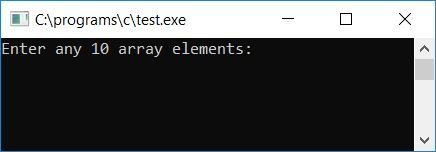Provide any 10 elements for the array and press ENTER key to see all the even values or elements from the given 10 array elements as shown in the second snapshot given here: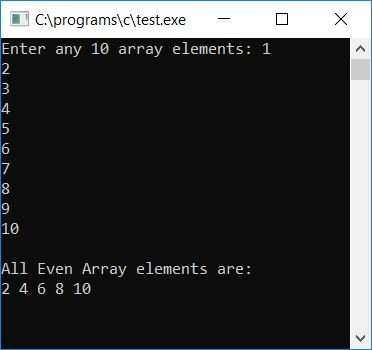These are some of the main steps used in above program:

• Receive any 10 array elements
• Create for loop starts from 0 to 9
• Inside the for loop, check whether the current element is an even number or not
• If it is an even number, print it out and continue to check for the next array element until all the 10 elements gets checked and printed
• In this way, we will see all the even array elements as output

Now let's modify the above program to create another array say b[] that will hold all the even elements of the original array. Therefore to print all the even elements of the given array, just print the array say b[] that holds all the even array elements. Here is the program:

```// Write a program in C to read 10 values in
// an integer array and print all even values
// -----------codescracker.com-----------

#include<stdio.h>
#include<conio.h>
int main()
{
int arr, i, b, j=0, count=0;
printf("Enter any 10 array elements: ");
for(i=0; i<10; i++)
scanf("%d", &arr[i]);
for(i=0; i<10; i++)
{
if(arr[i]%2==0)
{
b[j] = arr[i];
count++;
j++;
}
}
printf("\n\nEven elements are:\n");
for(i=0; i<count; i++)
{
if(i==(count-1))
printf("%d", b[i]);
else
printf("%d, ", b[i]);
}
getch();
return 0;
}```

Here is the first snapshot of the sample run: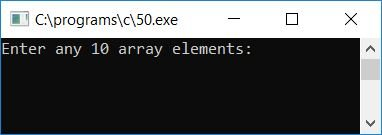Provide any 10 elements for the array and press ENTER key to see the output as shown in the second snapshot given here: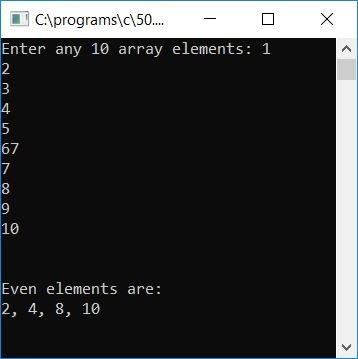Here are some of the main steps used in above program:

• Receive any 10 array elements
• Create a for loop starts from 0 to 9
• Inside the for loop, check whether the current element is an even number or not
• If it is an even number, then place this element to the second array say array b[]
• Indexing starts for array b[] will be from 0, and each time increment the index value with 1 whenever the element is found as even number (in original array) and placed inside this array
• After checking and placing each and every original array elements (if found as even number) inside the second array say b[], come out from the for loop
• Now print the value of second array say b[], that will hold all the even array elements of the original array

Here is the modified version of the above program. In this program user is also allowed to provide size for the array.

```// Write a program in C to allow user to enter
// the size for the array and
// enter array elements of that given size
// as input
// to print all all the even elements
// as output
// -----------codescracker.com-----------

#include<stdio.h>
#include<conio.h>
int main()
{
int arr, i, b, j=0, count=0, size;
printf("Enter the size for array: ");
scanf("%d", &size);
printf("Enter any 10 array elements: ");
for(i=0; i<size; i++)
scanf("%d", &arr[i]);
for(i=0; i<size; i++)
{
if(arr[i]%2==0)
{
b[j] = arr[i];
count++;
j++;
}
}
printf("\nAll Even elements are:\n");
for(i=0; i<count; i++)
{
if(i==(count-1))
printf("%d", b[i]);
else
printf("%d, ", b[i]);
}
getch();
return 0;
}```

Here is the final snapshot of the above program: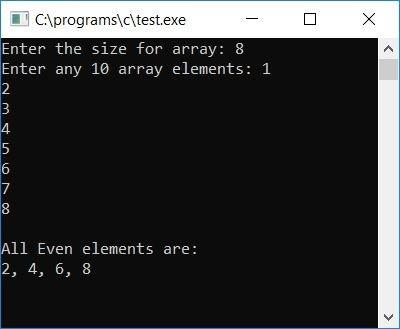Tools
Calculator